• 设计一个按照优先级调度算法实现处理机调度的程序 一、优先级调度算法实现处理机调度的程序设计提示如下： ( 1 ) 假设系统n个进程，每个进程用一个进程控制块（PCB）来代表。进程控制块的格式如下表所示，且参数...
文章目录设计一个按照优先级调度算法实现处理机调度的程序一、优先级调度算法实现处理机调度的程序设计提示如下：二、程序中使用的数据结构及主要符号说明三、程序流程图四、参考代码五、运行结果六、总结
设计一个按照优先级调度算法实现处理机调度的程序
一、优先级调度算法实现处理机调度的程序设计提示如下：

( 1 )  假设系统有n个进程，每个进程用一个进程控制块（PCB）来代表。进程控制块的格式如下表所示，且参数意义也相同。进程的优先数、到达时间和估计运行时间由用户程序任意设定，且优先数越低，优先级越高。调度时，总是选择优先级最高的进程运行。
（2）为了调度方便，设计一个指针指向就绪队列的第一个到达进程。另外再设一个当前运行进程指针，指向当前正运行的进程。
（3）处理机调度时，总是选择已经到达队列的优先级最高的进程运行。为了采用动态优先级调度，进程每运行一次，其优先级就减1。
（4）由于本题目是模拟实验，所以对被选中的进程并不实际启动运行，而只是执行如下操作：
1）优先数加1；
2）估计运行时间减1；
3）输出当前运行进程的名字。
用这三个操作来模拟进程的一次运行。
（5）进程运行一次后，应判断该进程的剩余运行时间是否为0，若不为0，且其优先级低于就绪队列的其他进程的优先级，则选择一个高优先级进程抢占CPU运行，若该进程的剩余运行时间为0，则将该进程的状态置为完成状态“C”，并撤出就绪队列。
（6）若就绪队列不为空，则重复上述的步骤（4）和（5）直到所有进程都运行完为止。
（7）在所设计的调度程序中，应包含显示或打印语句，以便显示或打印每次选中进程的名称及运行一次后进程的变化以及就绪队列中各进程排队情况。

二、程序中使用的数据结构及主要符号说明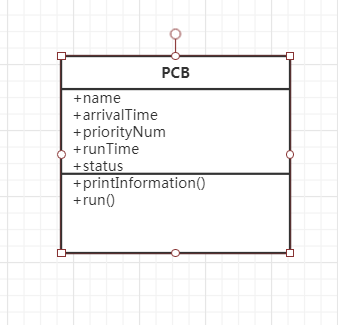模拟PCB进程控制块设置了如上数据结构，其中name是进程名，arrivalTime是到达时间，priorityNum是优先级数，runTime是运行时间，status是进程状态。
其中：
name为用户程序自定义的
priorityNum为1~10的随机数
arrivalTime和runTime为1~5的随机数
status的值有三种：Ready，Working，End，分别代表就绪状态，运行状态和终止状态。

三、程序流程图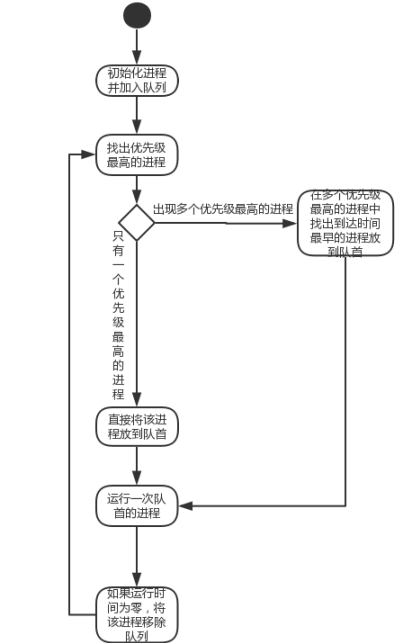四、参考代码
PCB类：
package pcb;

public class PCB {
private String name;//进程名字
private int arrivalTime;//到达时间,1~5的随机数
private int priorityNum;//优先级，1~10的随机数
private int runTime;//运行时间，1~5的随机数
private String status = "Ready";//进程状态

public PCB(String name) {
super();
this.name = name;
this.setArrivalTime(arrivalTime);
this.setPriorityNum(priorityNum);
this.setRunTime(runTime);
//this.printInformation();
}

//打印PCB信息
public void printInformation() {
System.out.println(this.getName()+"\t"+this.getArrivalTime()+"\t"+
this.getRunTime()+"\t"+this.getPriorityNum()+"\t"+this.getStatus());
}

//	public PCB(String name, int arrivalTime, int priorityNum, int runTime) {
//	super();
//	this.name = name;
//	this.arrivalTime = arrivalTime;
//	this.priorityNum = priorityNum;
//	this.runTime = runTime;
//	this.printInformation();
//}

//进程运行
public void run() {
this.priorityNum++;
this.runTime--;
}

public String getName() {
return name;
}

public void setName(String name) {
this.name = name;
}

public int getArrivalTime() {
return arrivalTime;
}

public void setArrivalTime(int arrivalTime) {
arrivalTime = (int) (Math.random()*5+1);
this.arrivalTime = arrivalTime;
}

public int getPriorityNum() {
return priorityNum;
}

public void setPriorityNum(int priorityNum) {
priorityNum = (int) (Math.random()*10+1);
this.priorityNum = priorityNum;
}

public int getRunTime() {
return runTime;
}

public void setRunTime(int runTime) {
runTime = (int) (Math.random()*5+1);
this.runTime = runTime;
}

public String getStatus() {
return status;
}

public void setStatus(String status) {
this.status = status;
}
}


Priority 类：
package pcb;

import java.util.Iterator;
import java.util.LinkedList;
import java.util.Queue;

public class Priority {
public static void getMax(Queue<PCB> queue) {
//找出优先级最高（优先级数最小）的进程
int minPriorityNum = 100;
int minArrivalTime = 100;
for(PCB q : queue ) {
if(q.getPriorityNum() < minPriorityNum) {
minPriorityNum = q.getPriorityNum();
}
}
//找出优先级最高且到达时间最早的进程
for(PCB q : queue ) {
if(q.getPriorityNum() == minPriorityNum && q.getArrivalTime() < minArrivalTime) {
minArrivalTime = q.getArrivalTime();
}
}

PCB first = queue.peek();
for(int i = 0;i < queue.size();i++) {
while(queue.peek().getPriorityNum() > minPriorityNum) {
queue.offer(queue.peek());
queue.poll();
}
PCB q =  ((LinkedList<PCB>) queue).get(i);

if(!q.equals(first)) {
if((q.getPriorityNum() == queue.peek().getPriorityNum()) && q.getArrivalTime() < queue.peek().getArrivalTime()) {
while(queue.peek().getArrivalTime() != minArrivalTime) {
queue.offer(queue.peek());
queue.remove(queue.peek());
queue.remove(0);
//i--;
}
}else {
continue;
}
}else {
continue;
}
}
}

public static void main(String[] args) {
System.out.println("名字\t"+"到达时间\t"+"运行时间\t"+"优先级数\t"+"状态");
Queue<PCB> queue = new LinkedList<PCB>();
//		queue.offer(new PCB("a1",1,9,9));
//		queue.offer(new PCB("a2",2,5,10));
//		queue.offer(new PCB("a3",5,7,10));
//		queue.offer(new PCB("a4",2,4,6));
queue.offer(new PCB("a1"));
queue.offer(new PCB("a2"));
queue.offer(new PCB("a3"));
//		queue.offer(new PCB("a4"));
//		queue.offer(new PCB("a5"));
//		queue.offer(new PCB("a6"));
//		queue.offer(new PCB("a7"));
//		queue.offer(new PCB("a8"));
//		queue.offer(new PCB("a9"));
//		queue.offer(new PCB("a10"));
//		queue.offer(new PCB("a11"));
//		queue.offer(new PCB("a12"));
//		queue.offer(new PCB("a13"));
//		queue.offer(new PCB("a14"));
//		queue.offer(new PCB("a15"));
//		queue.offer(new PCB("a16"));

//		System.out.println("=======================================");

while(!queue.isEmpty()) {
//把优先级最高（优先级数小）的进程调到队首
if(queue.size() > 1) {
getMax(queue);
}

if(!queue.isEmpty()) {
//遍历队列，设置进程状态
for(PCB q : queue) {
if(q.equals(queue.peek())) {
//队首
q.setStatus("Working");
}else {
//非队首
if(q.getStatus().equals("Working")) {
q.setStatus("Ready");
}
}
//打印信息
q.printInformation();
}
//进程运行
queue.peek().run();
}

for(PCB q : queue) {
if(q.getRunTime() == 0) {
q.setStatus("End");
}

if(q.getStatus().equals("End")) {
System.out.println();
//打印信息
q.printInformation();
System.out.print("==============="+q.getName()+"进程结束================");
queue.poll();
break;
}
}
System.out.println();
}
}
}


五、运行结果
1.第一次运行结果：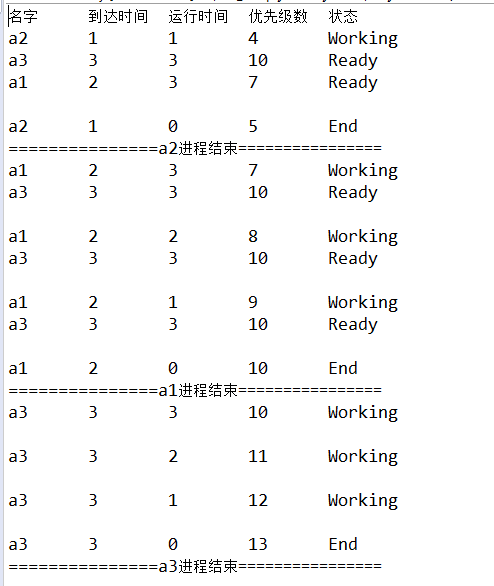2.第二次运行结果：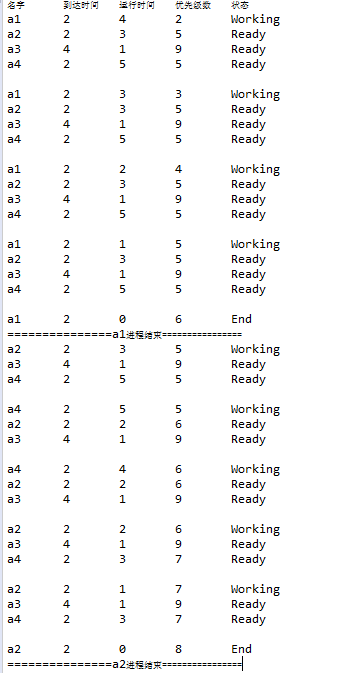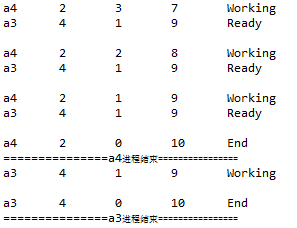六、总结

本实验是模拟优先级调度算法实现处理机调度的程序，实验中假设所有创建的进程都已在内存中，即一个等待运行的队列中，每次都将优先级最高的进程放到队首，然后每运行一次进程就下降一个优先权再进行优先权比较 ，如果优先级相同则比较到达时间，将优先级最高且到达时间最早的进程放到队首。若出现优先权更高的进程，则立即停止当前执行，并将处理机分配给新到的优先权最高的进程。
本程序测试过程中加入了32个进程，程序运行稳定且结果正确，说明了程序的鲁棒性较好。


展开全文JAVA 优先级调度 操作系统
• 题目一：设计一个按照时间片轮转法实现处理机调度的程序 时间片轮转法实现处理机调度的程序设计提示如下： （1）假设系统n个进程，每个进程用一个进程控制块（PCB）来代表。进程控制块的格式如下表所示，且参数...
要求：书写课程设计报告，报告中应该包含如下内容：
（1）课程设计题目及内容
题目一：设计一个按照时间片轮转法实现处理机调度的程序
时间片轮转法实现处理机调度的程序设计提示如下：
（1）假设系统有n个进程，每个进程用一个进程控制块（PCB）来代表。进程控制块的格式如下表所示，且参数意义也相同。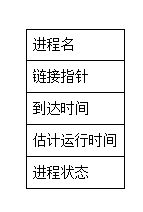（2）按照进程到达的先后顺序排成一个循环队列，设一个队首指针指向第一个到达进程的首址。另外再设一个当前运行进程指针，指向当前正运行的进程。
（3）执行处理机调度时，首先选择队首的第一个进程运行。
（4）由于本题目是模拟实验，所以对被选中的进程并不实际启动运行，而只是执行如下操作：1）估计运行时间减1；
2）输出当前运行进程的名字。
用这两个操作来模拟进程的一次运行。
（5）进程运行一次后，以后的调度则将当前指针依次下移一个位置，指向下一个进程，即调整当前运行指针指向该进程的链接指针所指进程，以指示应运行进程，同时还应判断该进程的剩余运行时间是否为0，若不为0，则等待下一轮的运行，若该进程的剩余运行时间为0，则将该进程的状态置为完成状态“C”，并退出循环队列。
（6）若就绪队列不为空，则重复上述的步骤（4）和（5）直到所有进程都运行完为止。
（7）在所设计的调度程序中，应包含显示或打印语句，以便显示或打印每次选中进程的名称及运行一次后队列的变化情况。
（2）程序中使用的数据结构及主要符号说明
int PCBNum;		//总进程数
#define Maxsize 50	//最大进程数
int t_time = 0;		//系统时间
typedef struct PNode
{
char name; //定义进程名，并分配空间
int Arr_Time; //定义到达时间
int ready_Time; //定义运行时间
char state; //定义进程状态R(就绪)/F(运行)/C(完成)
}PNode;
typedef struct
{
PNode *data;
PNode* ready;
int datafront, datarear;			//数据存储队列队首队尾
int readyfront, readyrear;	//就绪队列队首队尾
}SqPCB;

（3）程序流程图和带有注释的源程序
程序流程图：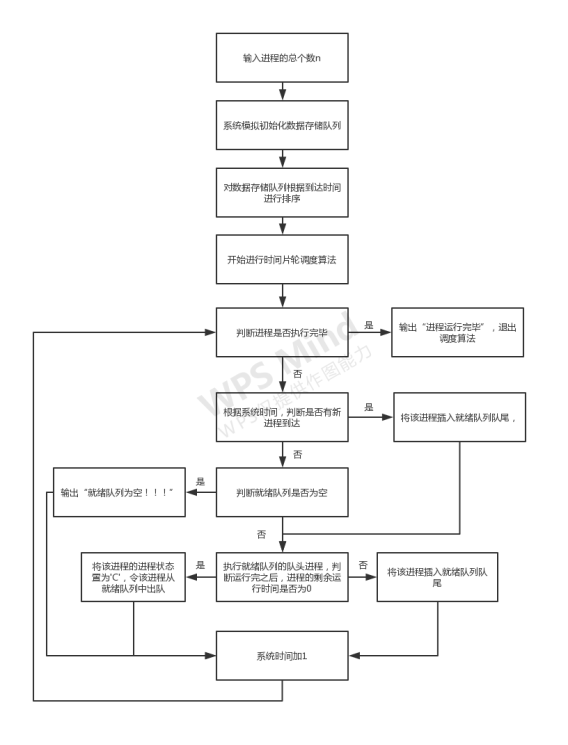源程序：
#include<iostream>
#include<time.h>
#include<string>
#include<string.h>
using namespace std;
int PCBNum;		//总进程数
#define Maxsize 50	//最大进程数
int t_time = 0;		//系统时间
typedef struct PNode
{
char name; //定义进程名，并分配空间
int Arr_Time; //定义到达时间
int ready_Time; //定义运行时间
char state; //定义进程状态R(就绪)/F(运行)/C(完成)
}PNode;
typedef struct
{
PNode *data;
PNode* ready;
int datafront, datarear;			//数据存储队列队首队尾
int readyfront, readyrear;	//就绪队列队首队尾
}SqPCB;
void lnitPCB(SqPCB& H)//初始化就绪队列
{
cout << "请输入总进程个数:";
cin >> PCBNum; ///进程总个数
cout << endl;
int Num = PCBNum;
H.datafront = 0;		//初始化数据存储队列队首
H.datarear = 0;			//初始化数据存储队列队尾
H.readyfront = 0;	//初始化就绪队列队首
H.readyrear = 0;	//初始化就绪队列队尾
H.data = (PNode*)malloc(Maxsize * sizeof(PNode));
H.ready = (PNode*)malloc(Maxsize * sizeof(PNode));
char ch = { 'A' };
PNode p;
srand((unsigned)time(NULL));
cout << "总进程个数为" << PCBNum << "个，请输入各个进程的名字(由于是模拟程序，到达时间和运行时间由随机数生成)" << endl;
while (Num--)
{
strcpy_s(p.name, ch);
ch = ch + 1;
p.Arr_Time = rand() % 8 + 1;
p.ready_Time = rand() % 10 + 1;
p.state = 'R';
H.data[H.datarear] = p;
H.datarear = (H.datarear + 1) % Maxsize;
}
cout << endl;
}
void show(SqPCB& H)
{
cout << "进程信息" << endl;
int i = H.datafront;
while(i!=H.datarear)
{
cout << "进程名:" << H.data[i].name
<< "\t到达时间:" << H.data[i].Arr_Time
<< "\t运行时间:" << H.data[i].ready_Time
<< "\t进程状态:" << H.data[i].state << endl;
i = (i + 1) % Maxsize;
}
cout << endl;
}
void showready(SqPCB& H)//打印就绪队列
{
cout << "就绪队列内进程信息(按处理机调度顺序)" << endl;
int i = H.readyfront;
if (H.readyfront == H.readyrear)
{
cout << "就绪队列为空！！！";
}
while (i != H.readyrear)
{
cout << "进程名:" << H.ready[i].name
<< "\t到达时间:" << H.ready[i].Arr_Time
<< "\t运行时间:" << H.ready[i].ready_Time
<< "\t进程状态:" << H.ready[i].state << endl;
i = (i + 1) % Maxsize;
}
cout << endl;
}
void sort(SqPCB& H)
{
for (int i = 0; i < PCBNum; i++)
{
for (int j = 0; j < PCBNum - 1 - i; j++)
{
if (H.data[j].Arr_Time > H.data[j + 1].Arr_Time)
{
PNode temp;
strcpy(temp.name, H.data[j].name);
temp.Arr_Time = H.data[j].Arr_Time;
temp.ready_Time = H.data[j].ready_Time;
strcpy(H.data[j].name, H.data[j + 1].name);
H.data[j].Arr_Time = H.data[j+1].Arr_Time;
H.data[j].ready_Time = H.data[j+1].ready_Time;
strcpy(H.data[j+1].name, temp.name);
H.data[j + 1].Arr_Time = temp.Arr_Time;
H.data[j+1].ready_Time = temp.ready_Time;
}
}
}
cout << "***********************************************************************" << endl;
cout << "排序之后的进程运行顺序：" << endl;
show(H);
}
void SJP_Simulator(SqPCB& H)
{
int* flag = new int[PCBNum];
int x = 0;
int o = H.datarear - H.datafront;
while (x!=PCBNum)//如果进程都执行完毕则结束循环
{
while (o)//判断是否有新的进程同时到达
{
if (t_time >= H.data[H.datafront].Arr_Time && H.datafront != H.datarear)//判断是否有新的进程到达
{

flag[H.datafront] = 1;
PNode temp;
strcpy(temp.name, H.data[H.datafront].name);		//就绪队列入队
temp.Arr_Time = H.data[H.datafront].Arr_Time;		//用temp结点存储数据
temp.ready_Time = H.data[H.datafront].ready_Time;
temp.state = H.data[H.datafront].state;
H.ready[H.readyrear] = temp;					//进程进入就绪队列队尾
H.readyrear = (H.readyrear + 1) % Maxsize;		//队尾后移
H.datafront++;										//数据存储队列队首后移
}
o--;
}
o = H.datarear - H.datafront;
if (H.readyfront != H.readyrear)//就绪队列不为空
{
if (H.ready[H.readyfront].ready_Time != 1)
{
cout << "--------------------------------系统时间为：" << t_time << "---------------------------------------" << endl;
cout << "当前运行的进程为:" << H.ready[H.readyfront].name << endl;
cout << "该进程时间片用完后运行时间由" << H.ready[H.readyfront].ready_Time << "变为" << H.ready[H.readyfront].ready_Time - 1 << endl;
H.ready[H.readyfront].ready_Time--;//运行时间-1
H.ready[H.readyfront].state = 'F';//更改进程状态为运行状态"F"
showready(H);
H.ready[H.readyfront].state = 'R';//运行完之后更改进程状态为就绪状态"R"
H.ready[H.readyrear] = H.ready[H.readyfront];	//将运行完之后的进程插入就绪队列队尾
H.readyrear = (H.readyrear + 1) % Maxsize;		//就绪队列队尾后移
H.readyfront = (H.readyfront + 1) % Maxsize;	//就绪队列队首后移
}
else if (H.ready[H.readyfront].ready_Time == 1)
{
cout << "--------------------------------系统时间为：" << t_time << "---------------------------------------" << endl;
cout << "当前运行的进程为:" << H.ready[H.readyfront].name << endl;
cout << "该进程时间片用完后运行时间由" << H.ready[H.readyfront].ready_Time << "变为" << H.ready[H.readyfront].ready_Time - 1 << endl;
cout << "进程" << H.ready[H.readyfront].name << "执行完毕！！" << endl;
x++;
H.ready[H.readyfront].state = 'C';//进程状态置为完成状态"C"
H.ready[H.readyfront].ready_Time--;//运行时间-1
showready(H);
H.readyfront = (H.readyfront + 1) % Maxsize;	//令该进程出队
}

}
else
{
cout << "--------------------------------系统时间为：" << t_time << "---------------------------------------" << endl;
showready(H);
}
t_time++;//系统时间+1
}
cout << "--------------------------------全部进程运行完毕！！--------------------------------" << endl;
}
int main()
{
SqPCB A;
lnitPCB(A);			//数据初始化
show(A);			//打印
sort(A);			//排序
SJP_Simulator(A);	//时间片轮转法
}

（4）执行程序，并打印程序运行时的初值和运算结果
运行程序，输入总进程个数，程序自动模拟生成进程信息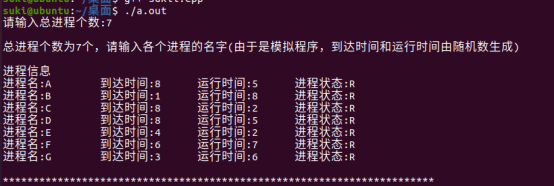结果进程全部运行成功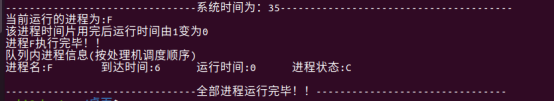（5）实验结果分析，实验收获和体会
进程信息系统模拟生成后会按照到达时间进行排序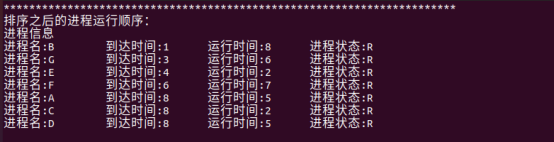当系统时间为0时，没有进程到达，此时就绪队列为空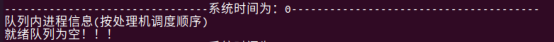当系统时间为1时，进程B到达，将进程C调入处理器运行，进程状态更改为‘F’（运行中），运行时间减1，运行完进程状态更改为‘R’（就绪）后调入就绪队列队尾，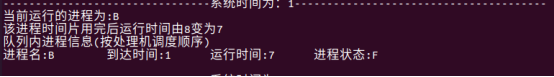当系统时间为3时，进程G到达，将进程G调入就绪队列队尾，此时队首为进程B，所以进程B调入处理器运行，进程状态更改为‘F’，运行时间减1，运行完后进程状态更改为‘R’（就绪）调入就绪队列队尾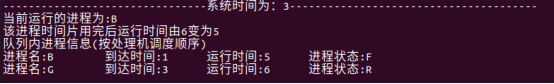当系统时间为4时，此前因为进程B运行完，调入就绪队列队尾，进程E到达，将进程E调入就绪队列队尾，此时队首为进程G，将进程G调入处理器运行，进程状态更改为‘F’，运行时间减1，运行完进程状态更改为‘R’（就绪）后调入就绪队列队尾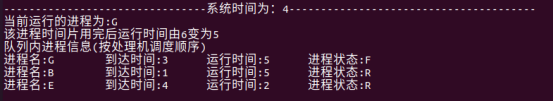当系统时间为10时，进程E运行时间减为0后，进程E运行完毕，将进程E的进程状态更改为‘C’（完成），然后将其调出队列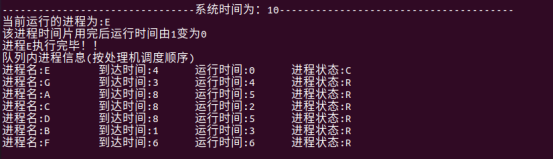当最后一个进程运行完毕后，全部进程运行完毕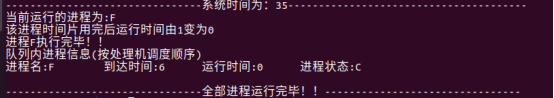收获和体会：
通过这次实验，加深了对时间片轮法的理解，对于就绪态，运行态的转换的认识更加深刻，同时对于数据结构队列的知识又复习了一遍，实验中出现了许多问题，如数组越界，内存泄漏，字符串string类的使用，对于这些问题又重新温习了一边，对于C++的使用更加熟练了。


展开全文c++ 操作系统
• 操作系统课程设计：设计一个按照时间片轮转法实现处理机调度的程序 一：时间片轮转法实现处理机调度的程序设计提示如下： （1） 假设系统n个进程，每个进程用一个进程控制块（PCB）来代表。进程控制块的格式如下表...
操作系统课程设计：设计一个按照时间片轮转法实现处理机调度的程序
一：时间片轮转法实现处理机调度的程序设计提示如下：
（1）
假设系统有n个进程，每个进程用一个进程控制块（PCB）来代表。进程控制块的格式如下表所示，且参数意义也相同。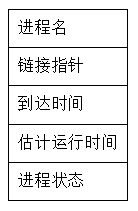（2）按照进程到达的先后顺序排成一个循环队列，设一个队首指针指向第一个到达进程的首址。另外再设一个当前运行进程指针，指向当前正运行的进程。
（3）执行处理机调度时，首先选择队首的第一个进程运行。
（4）由于本题目是模拟实验，所以对被选中的进程并不实际启动运行，而只是执行如下操作：1）估计运行时间减1；
2）输出当前运行进程的名字。
用这两个操作来模拟进程的一次运行。
（5）进程运行一次后，以后的调度则将当前指针依次下移一个位置，指向下一个进程，即调整当前运行指针指向该进程的链接指针所指进程，以指示应运行进程，同时还应判断该进程的剩余运行时间是否为0，若不为0，则等待下一轮的运行，若该进程的剩余运行时间为0，则将该进程的状态置为完成状态“C”，并退出循环队列。
（6）若就绪队列不为空，则重复上述的步骤（4）和（5）直到所有进程都运行完为止。
（7）在所设计的调度程序中，应包含显示或打印语句，以便显示或打印每次选中进程的名称及运行一次后队列的变化情况。
二：程序中使用的数据结构以及主要符号说明；
（1）程序中时间片轮转的实现采取了链式队列的数据结构。需要分别创建一个节点结
构 QueueNode 以及一个队列结构Queue。QueueNode中有数据以及指针（题目
中的链接指针）.队列中包括队头head以及队尾指针tail。
（2）一个进程结构 process 结构里有：进程名字：processname、到达时间：arrivetime
运行时间：runtime、进程状态：status，将所有的进程放入一个节点结构数组中
（sturct process a[]）
（3）程序中的主要符号说明：
n:进程个数    num:运行的次数    m:队列中的实时进程数量
三：程序流程图和带有注释的源程序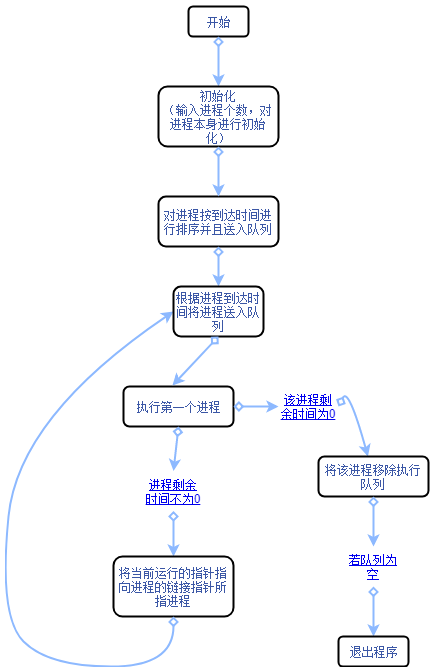源程序：
#include <iostream>
#include<time.h>
#include<stdlib.h>
#define N 100
using namespace std;
typedef string datatype;//进程的名字类型
typedef int intt;
typedef struct process//进程结构
{
datatype processname;//进程名字
intt arrivetime;//到达时间
intt runtime;//运行时间
datatype status;//进程状态
};
typedef struct QueueNode//定义一个节点
{
process data;
QueueNode* next;
}QueueNode;
typedef struct Queue//定义队列
{
QueueNode* head;//队头
QueueNode* tail;//队尾
size_t size;//记录当前队列里的元素个数
}Queue;
void QueueInit(Queue *&q)//队列初始化
{
q = (Queue *)malloc(sizeof(Queue));
q->head = NULL;
q->tail = NULL;
q->size = 0;
}
void QueuePush(Queue *&q, process data)//入队
{
QueueNode* newnode = new QueueNode;//创建新的节点
newnode->data= data;//节点数据等于要入队的数据
newnode->next = NULL;//节点的链接指针设为空
if (q->size == 0)//将节点送入队列
{
q->head = newnode;
q->tail = newnode;
q->size++;
}
else
{
q->tail->next = newnode;
q->tail = newnode;
q->size++;
}
}
bool QueuePop(Queue *&q)//出队
{
if (q->size == 0)
{
exit(1);
}
else if (q->size == 1)
{
q->head = NULL;
q->tail = NULL;
q->size--;
}
else
{
QueueNode* p = q->head;//领队列的头指针指向一个新的节点
p->data= q->head->data;//该节点的数据等于要出队的数据
q->head = p->next;//另头指针指向下一个节点
q->size--;
free(p);
}
}
int Queuesize(Queue*& q)//返回队列长度
{
return q->size;
}
void ShowQueue(Queue*& q)//展示队列中的顺序（即执行顺序）
{
QueueNode* p = q->head;
for (int i = 0; i < Queuesize(q); i++)
{
if (i == 0)
{
cout << "进程" << q->head->data.processname << "执行  ";
}
else
{
p = p->next;
cout << "进程" << p->data.processname << "等待  ";
}
}
}
void bubbleinsert(struct process  a[] ,int len)//把进程的到达时间按照冒泡排序。
{
struct process b[N] ;
for (int i = 0; i < len; i++)
{
for (int j = i + 1; j < len; j++)
{
if (a[j].arrivetime < a[i].arrivetime)
{
b= a[i];
a[i] = a[j];
a[j] = b;
}
}
}
}
void change(struct process a[],int n)//将已经运行完成的进程移出data
{
int i = 0;
int j = 0;
while (j < n)//去除a[]中 a[].runtime=0的进程。
{
if (a[j].runtime != 0)
{
a[i] = a[j];
i++;
}
j++;
}
}
int main()
{
srand((unsigned)time(NULL));
int n = 0, m = 0, pnum = 0;
int  flag;
struct process p[N] ;
Queue *q;
QueueInit(q);
n = rand() % 10 + 1;
cout << "进程个数：" << n << endl;
cout << "\n进程初始化（输入各个进程的数据）：\n";
for (int i = 0; i < n; i++)//进程初始化
{
p[i].processname='a'+i;
p[i].arrivetime=rand()%15;
p[i].runtime=rand()%6+1;
p[i].status = "P";
}
bubbleinsert(p, n);//把进程按照到达时间顺序排序
cout << "进程名字" << "\t" << "到达时间" << "\t" << "需运行时间" << "\t" << "进程状态" << endl;
for (int i = 0; i < n; i++)//进程初始化
{
cout << "   " << p[i].processname << " \t\t"
<< "   " << p[i].arrivetime << " \t\t"
<< "   " << p[i].runtime << " \t\t"
<< "   " << p[i].status << endl;
}
m = n;
for (int i = 0;; i++)//时间
{
flag =0;//表示队列中的个数没有变化
cout << "\n时间" << i << ": " << endl;
n = m;
for (int j = 0; j < n; j++)
{
if (p[j].arrivetime == i)//如果到达时间与时间相同
{
QueuePush(q, p[j]);//入队
cout << "进程" << p[j].processname << "到达并开始执行" << endl;
pnum++;
}
}
if (Queuesize(q) != 0)//更新process中的数据
{
for (int k = 0; k < n; k++)
{
if (p[k].processname == q->head->data.processname)
{
p[k].runtime--;
if (p[k].runtime == 0)
{
p[k].status = 'C';
m--;
flag = 1;
}
}
}
//处理队列中的数据
ShowQueue(q);//展示执行、等待顺序
q->head->data.runtime--;//头指针指向的进程处理时间-1
if (q->head->data.runtime == 0)
{
q->head->data.status = "C";//表示进程完成
cout << "进程" << q->head->data.processname << "处理完毕,移出队列！" << endl;
QueuePop(q);//出队
}
else
{
process p1;
p1 = q->head->data;
QueuePop(q);//出队
QueuePush(q, p1);//重新入队
}
cout << "\n运行" << i << "次后队列中的情况" << endl;
for (int t2 = 0; t2 < pnum; t2++)
{
cout << "第" << t2 + 1 << "个进程：" << "进程名：" << p[t2].processname
<< "\t剩余处理时间：" << p[t2].runtime << "\t进程状态：" << p[t2].status << endl;

}
if (flag == 1)
{
pnum--;//队列中的进程数量减1
}

change(p, n);
if (m == 0)// 所有进程都运行完毕
{
cout << "\n全部进程都已处理完毕，队列中无元素，结束程序";
break;
}
}
else
{
cout << "\n无进程到达、无进程运行" << endl;
}
}
}


四：执行程序名，并打印程序运行时的初值和运算结果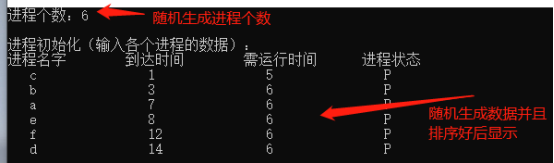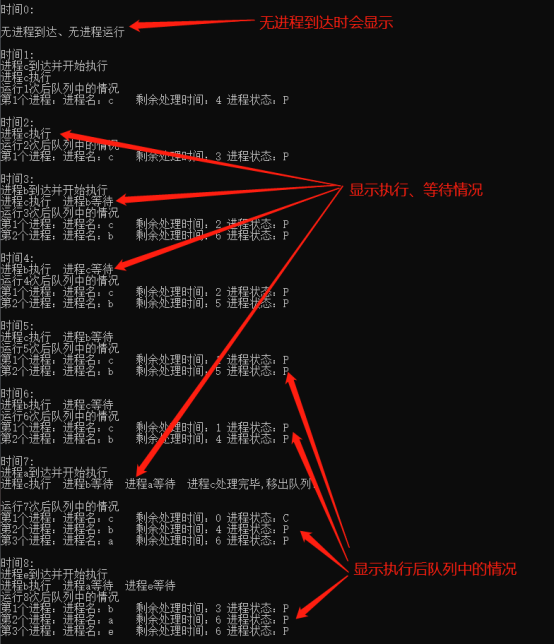五：实验结果分析，实验收获和体会
由最终的实验结果得：
输入进程数据后，会自动按照进程的到达顺序将进程排好序并且输出；
每个时间片运行一次后，当前运行的进程剩余时间-1，并且会将运行的结果输出（如**进程处理完毕，移出队列！），并且现实该次运行以后，队列中进程的信息；直到所有进程全部运行完毕。
实验总结：
一开始的时候，我把进程结构process单独写出来了，没有与QueueNode中的data进行关联，导致在进程入队出队的时候用到process结构数组的过于多，使队列存存在感很低，滥用了内存。所以做的第一个改进就是把process和QueueNode中的data进行关联（process *data)，这样就能使所有的数据直接全部压入队列中，方便出入队列，减少了内存的开销。
实验中我使用了一个for循环代表时间的增加，每一次时间片内运行队列中的第一个进程(运行完之后如进程运行完毕则移出队列，反之则将该进程放到队尾）并且接收该时间到达的新进程将其排到队尾从而实现“时间片轮转算法”，然后用一个结构数组来表示所有进程的数据。但是结构数组和队列没有关联，导致运行结束的进程出队之后，相应的数组中的数据没有进行更新反而还留着。就出现了在下一次轮转的时候原本结束的进程依然出现在队列中，而且runtime=-1并且无限循环。所以我设置了一个删除数组元素的函数change（struct process a[],int n)，这样运行完毕的进程相应的数据也会从process的结构数组中删除。
这次实验让我温习了结构数组、以及链式队列的相关知识点以及操作。并让我很深刻的体会了时间片轮转算法的运行过程。实验中的时间复杂度还有待改善：在“轮转的时候时间复杂度为：O(n),但是对进程的到达时间排序的时候本实验用的是冒泡排序，时间复杂度为：o(n^2),.可以采取同样稳定的时间复杂度为nlog(n)的归并排序


展开全文• 题目一：设计一个按照时间片轮转法实现处理机调度的程序 虽然该文内容的是题目二，但是题目一和题目二也就调度方式不一样，其他都是完全相同的，也可以作为参考。 题目二：设计一个按照优先级调度算法实现处理机调度...
操作系统课设
题目一：设计一个按照时间片轮转法实现处理机调度的程序
虽然该文内容的是题目二，但是题目一和题目二也就调度方式不一样，其他都是完全相同的，也可以作为参考。
题目二：设计一个按照优先级调度算法实现处理机调度的程序
优先级调度算法实现处理机调度的程序设计提示如下：
（1）	假设系统有n个进程，每个进程用一个进程控制块（PCB）来代表。进程控制块的格式如下表所示，且参数意义也相同。进程的优先数、到达时间和估计运行时间由用户程序任意设定，且优先数越低，优先级越高。调度时，总是选择优先级最高的进程运行。
进程名
链接指针
进程的到达时间
进程的优先级
估计运行时间
进程状态
（2）	为了调度方便，设计一个指针指向就绪队列的第一个到达进程。另外再设一个当前运行进程指针，指向当前正运行的进程。
（3）	处理机调度时，总是选择已经到达队列的优先级最高的进程运行。为了采用动态优先级调度，进程每运行一次，其优先级就减1。
（4）	由于本题目是模拟实验，所以对被选中的进程并不实际启动运行，而只是执行如下操作：1）优先数加1；
2）估计运行时间减1；
3）输出当前运行进程的名字。
用这三个操作来模拟进程的一次运行。
（5）	进程运行一次后，应判断该进程的剩余运行时间是否为0，若不为0，且其优先级低于就绪队列的其他进程的优先级，则选择一个高优先级进程抢占CPU运行，若该进程的剩余运行时间为0，则将该进程的状态置为完成状态“C”，并撤出就绪队列。
（6）	若就绪队列不为空，则重复上述的步骤（4）和（5）直到所有进程都运行完为止。
（7）	在所设计的调度程序中，应包含显示或打印语句，以便显示或打印每次选中进程的名称及运行一次后进程的变化以及就绪队列中各进程排队情况。
数据结构

每一个进程控制块都有多个数据项，可以用结构体将多个进程连成链表
struct pid {
string name;//进程名
pid* next;//链接指针
int arrive_time = 0;//到达时间
int grade = 0;//进程的优先级数
int run_time = 0;//预计运行时间
string state;//进程状态
};

需要一个指针来指向当前就绪列表里面优先级最高的进程（也就是当前运行的进程）

还需要一个指针来指向优先度最高的进程的上一个节点（用于进程执行完成，进程移出就绪队列）

还需要设置一个全局变量来代表系统运行的时间

函数

创建链表函数
创建一个头指针，尾指针，数据项指针。
给头尾节点分配内存， 头节点的下一个节点为空，尾节点等于头节点。
然后用一个循环，里面给数据项指针分配空间，然后初始化数据项，再把数据项节点加到链表尾部，该节点成为新的尾节点。
结束后返回头指针。

展示运行后就绪列表函数
把头指针作为参数传进来。
利用到达时间和当前系统时间对比判断，输出当前时间已经进入就绪列表的进程的情况。
利用指针下一项为空做结束标志。

找到优先级最高的进程并执行函数（也就是修改数据和输出）
把头指针作为参数传进来。
将当前最高优先级指针设置为空。
利用循环，找出当前时间已经到达的就绪队列里面优先级最高的进程。
用指针下一项是否为空作为结束标志，结束了代表遍历了一遍链表。
刚进入的时候判断进程在当前时间是否到达就绪列表，如果到达并且当前最高优先级指针为空则用当前最高优先级指针指向它，
进程在当前时间都到达就绪列表的情况下，先看谁的优先级数更小，优先级数越小，进程优先级越大，如果指针指向的进程的优先级数更小，则用该进程节点替换掉就绪队列最高优先级指针中的内容。
如果两个判断语句都没有进入，那指针就指向下一个节点。
循环结束之后，就代表当前系统最高优先级的进程已经被找出来，修改数据。输出当前系统时间，系统时间+1。
判断当前最高优先级指针是否为空，不为空则调用展示运行后就绪列表函数把就绪列表中的进程进行输出，然后判断该进程的预计运行时间是否为0，为0则把最高优先级指针置为空，并把该进程节点从链表删除，（利用一开始设置的当前运行进程的前一个节点指针）
如果当前最高优先级指针为空则输出说明当前时间就绪列表内没有进程。

主函数
创建一个指针，接受调用创建链表函数的返回值。
利用while循环，用链表是否为空做判断，循环调用找到优先级最高的进程并执行函数。

源码
#include<iostream>
#include<ctime>
#include<string>
#include<stdlib.h>
using namespace std;

struct pid {
string name;//进程名
pid* next;//链接指针
int arrive_time = 0;//到达时间
int grade = 0;//进程的优先级
int run_time = 0;//预计运行时间
string state;//进程状态
};
pid* runing = new pid;//指向正在运行的进程的指针
pid* runing_last = new pid;//指向正在运行的进程的上一个进程的指针
int M = 0;//系统时间
pid* creat() {//初始化创建进程列表
pid* head, * end, * normal;
int a = 97;
int m;//进程的数目
srand((unsigned)time(NULL));
m = rand() % 5 + 1;//随机生成1-5个进程
head = new pid;//分配内存
end = head;
end->next = NULL;
while (m) {//运行m次，生成m个进程
normal = new pid;
normal->name = a;//初始化进程名
normal->arrive_time = rand() % 5 + 1;//进程到达时间随机初始化为1-10
normal->grade = rand() % 5 + 1;//进程优先级随机初始化为1-10
normal->run_time = rand() % 5 + 1;//进程预计运行时间随机初始化为1-5
normal->state = "就绪";//设置进程状态
end->next = normal;//将新的进程放在尾节点的进程后面
end = normal;//新的进程成为尾进程
m--;//自减，用来计数
a++;//用来起名，保证每一个进程名不同
}
end->next = NULL;//创建完成之后，将尾节点的下一个节点置为空
pid* p;
p = head;//将头节点传递给p指针
cout << "队列中的情况:" << endl;
cout << "进程名" << '\t' << "到达时间" << '\t' << "优先数" << '\t' << "估计运行时间" << '\t' << "进程状态" << endl;
while (p->next != NULL) {//输出初始化生成的进程数据
p = p->next;
cout << p->name << '\t' << p->arrive_time << '\t' << '\t' << p->grade << '\t' << p->run_time << '\t' << '\t' << p->state << endl;
}
cout << "-------------------------分割线--------------------------" << endl;
return head;//返回头指针
}
void display(pid* head) {
pid* p = head;//将头指针传给p指针
if (p->next == NULL) {//p指针指向的下一个节点为空则代表链表为空，
cout << "就绪队列中已经没有进程了！";
}
else {
cout << "运行完成后就绪队列中的情况:" << endl;
cout << "进程名" << '\t' << "到达时间" << '\t' << "优先数" << '\t' << "估计运行时间" << '\t' << "进程状态" << endl;
while (p->next != NULL) {
if (p->next->arrive_time <= M) {//链表不为空，则把链表内到达时间小于等于系统时间的进程输出
p = p->next;
cout << p->name << '\t' << p->arrive_time << '\t' << '\t' << p->grade << '\t' << p->run_time << '\t' << '\t' << p->state << endl;
}
else {
p = p->next;
}
}
cout << "-------------------------分割线--------------------------" << endl;
}
}
int run(pid* head) {
pid* p;
p = head;//将头指针传给p指针
M++;//M自增，代表系统时间加一
while (1) {//循环遍历整个链表，找出优先级最高的进程

if (p->next == NULL) {//如果p指向的下一个节点为空，则代表已经遍历了链表，退出循环
break;
}
if ((p->next->arrive_time <= M) && runing==NULL) {//进程到达时间小于等于当前系统时间，并且当前最高优先级指针为空则用指针指向该节点
runing = p->next;//用当前运行进程指针指向第一个符合条件的进程
runing_last = p;//用指针指向第一个符合条件的进程的上一个节点
p = p->next;
}

else if (runing!=NULL&&(p->next->arrive_time <= M) && (p->next->grade) < (runing->grade) ) {//按照优先数越小，优先级越高，并且只有当前最该优先级指针不为空才会进行优先级比较
runing = p->next;//如果p的下一个节点优先级更高，则用p的下一个节点内容替换当前系统运行指针的内容
runing_last = p;
p = p->next;//p等于p的下一个节点
}

else {
p = p->next;//如果上面都不符合，p也等于下一个节点

}
}

cout << "当前的时间为：" << M << endl;//输出当前系统时间
if (runing != NULL) {//如果当前运行进程指针不为空，则代表就绪列表中有进程，可以进行输出
cout << "当前运行的进程为：" << runing->name << "进程！" << endl;
runing->grade = (runing->grade) + 1;//优先级数加一
runing->run_time = (runing->run_time) - 1;//预计运行时间减一
runing->state = "运行";//状态变为运行态
if (runing->run_time == 0) {//判断进程是否进入完成态
cout << "进程" << runing->name << "已经运行完毕，退出就绪队列！" << endl;
runing->state = "C";//置进程的状态为完成态
runing_last->next = runing->next;//从链表中删除该节点
delete runing;//释放内存
runing = NULL;//节点删除之后，把当前最高优先级指针置为空
display(head);//调用函数，输出当前就绪队列中的情况
}
else {//如果没运行完成，则把进程的状态切换回就绪态，
display(head);
runing->state = "就绪";
}
}
else {//就绪队列中没有进程
cout << "当前就绪列表无进程!" << endl;
}

return 0;//返回0
}

void main() {
runing = NULL;//一开始把当前最高优先级指针置空
pid* p;//声明指针
p = creat();//创建链表，用指针接受返回的头指针
while (p->next!=NULL) {//利用循环，一直到链表中的进程全部退出链表才会结束循环
run(p);//调用函数，进行优先级调度

}
}

运行结果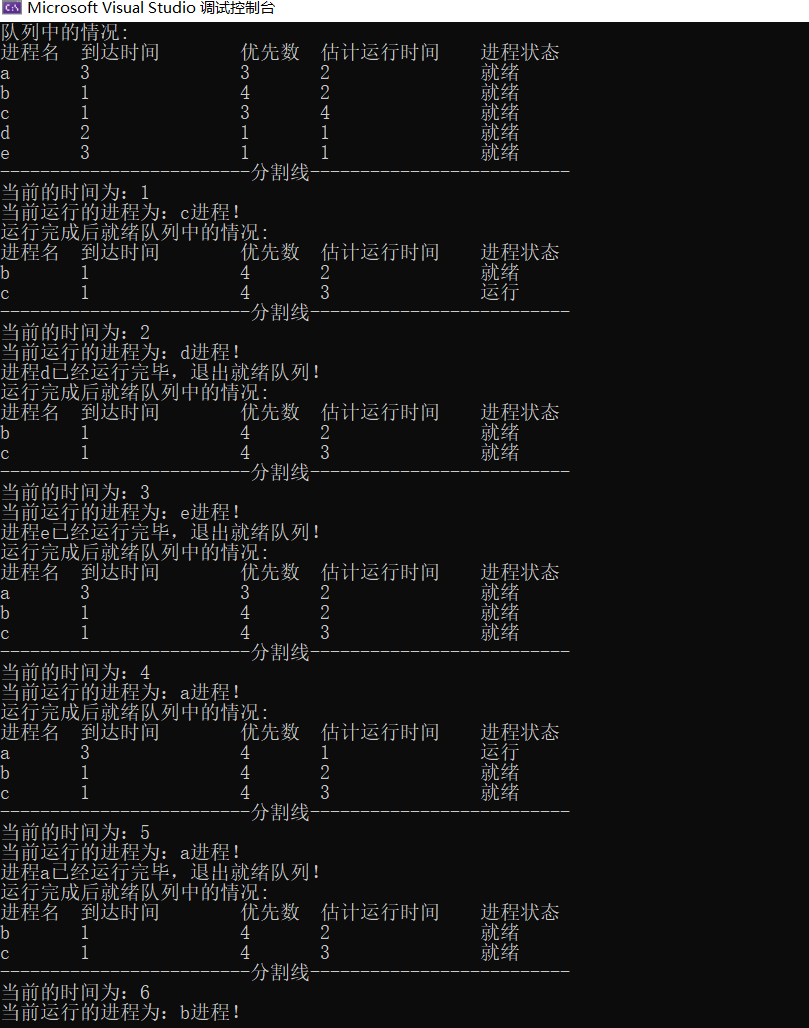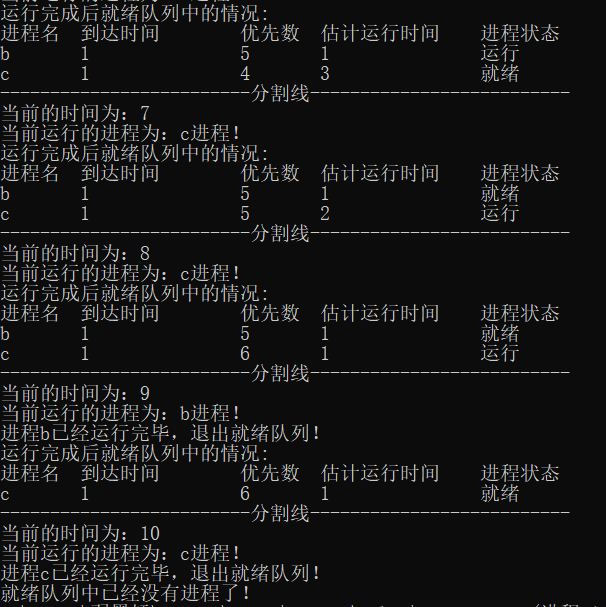注意事项
1.当前运行指针指向的进程优先级和就绪列表中的进程优先级相同的时候，运行当前指针指向的进程。（也就是不作交换）
2.注意到达时间，系统到了到达时间，进程才进入系统。
如果有错，可以指出啊！谢谢！啊哈哈哈！


展开全文操作系统 c++ 链表
• 1.假设系统n个进程，每个进程用一个进程控制块（PCB）来代表。进程控制块的格式如下表所示，且参数意义也相同。进程的优先数、到达时间和估计运行时间由用户程序任意设定，且优先数越低，优先级越高。调度时，总是...操作系统 多线程
• 碰到了一些问题，主要是事务处理的！！ 另外最近了考中程的想法，因为自己很想证明一下自己是能力学习的。本人不是计算机专业！
• 有一个处理单价为5角钱饮料的自动售货：若投入5角钱或1元钱的硬币，压下〖橙汁〗或〖啤酒〗的按钮，则相应的饮料就送出来。若售货没有零钱找，则一个显示〖零钱找完〗的红灯亮，这时在投入1元硬币并压下按钮后，...java
• 进程调度就是按照某种规则，从就绪队列中选择一个进程为其分配处理机。 那什么时候需要进行进程调度呢？ 有些时候是不能进行进程调度的： 中断的时候； 进程在操作系统内核程序临界区中，但是
• ## 处理机管理

千次阅读 2017-09-14 21:37:53
并发是指在逻辑上或宏观上的并行（或者说它们的执行在时间上重叠，一个程序的执行尚未结束，另一个程序的执行已经开始）；而并行操作才是真正的并行，或称为微观上的并行。2、并发程序执行的条件（Bernstein条件...操作系统 进程 线程
• MemoryHandler的性能问题: 如果target是StreamHandler的子类 上是严重的IO性能问题 是一个for调用handler， handler中的处理侧是 io.write(), io.flush() ...立马的flush到硬盘中，并多...但对多进程写同一个django logging python 多进程 服务器
• 最近在学习Nginx，偶然间发现官网一下翻译的原作者的一些文章不错，适合新手，于是调整了一下格式，转载在博客里，欢迎大家讨论。 ----------------------------------------------... 4、一个简单PHP站点配置 基
• 如何将一个有界面的正常app和一个或多个越狱插件.deb同时安装到手机上，这样...360应该也是监听和显示分开处理的，后台2个插件和一个守护进程在时时监听，前面是一个app。 界面的东西就记录用户的设置。插件就通插件
• 很少人意识到这些小型设备为了维持一个电话谈话需要每秒执行数百万次的计算，如果我们细看将语音电子信号转换为比特序列的模块，就会发现每20毫秒的输入语音就会被计算出一组语音参数然后传输到接收端，之后接收端...手机
• 要求学生设计一个模拟单处理机调度的算法，以巩固和加深处理机调度的概念。 二、 实验内容 处理机管理是操作系统中非常重要的部分。为深入理解进程管理部分的功能，设计几个调度算法，模拟实现处理机的调度。 FC...c++
• 一个线程是操作系统中对一个处理的 抽象。   创建新进程： （1）选择目标； （2）创建一个新的执行环境； （3）在执行环境中创建一个线程；   进程和线程的区别： 线程的创建和管理开销小，线程间 ...分布式
• ## 处理机调度FAQ

千次阅读 2013-09-01 20:53:11
也正因为中断与处理机调度之间密不可分的关系，我们将中断与处理机调度作为一个独立的完整章来讲授。读者应当注意，中断响应后系统首先处理中断事件，然后才考虑处理机的重新分派；当中断嵌套发生时，只有在返回至...linux
• 提要第三人称相机非常多种，今天要实现的一个第三人称射击游戏的相机。如果对相机控制不是很了解，建议看一下上一篇博文 FPS相机。控制思路鼠标控制yaw和pitch，添加一个distance变量来记录角色和相机之间的距离...unity3d
• 原问题描述： ...我想设定一个时间期限， 如果手机接收到电话时，就会自动的发给来电用户一个信息。 如何设置？ 解决方案： 你需要在 Activity 或者 Service中申明 PhoneStateListener： PhoneStateL移动开发 ANDROID
• 多处理通常来说分为3类： 共享内存的多处理，多个cpu共享同一个储存器，单向通信时间2-10ns 消息传递多计算机，每个储存器局部对应一个cpu，且只能被该cpu访问，这些cpu通过互联网网络发送多字消息...8.1 多处理机操作系统 读书笔记 分布式
• 实验一:处理机调度仿真(10学时) 实验内容: 在处理机三级调度系统中，编程仿真作业调度与进程调度。...为了使系统中各进程能有条不紊地工作，必须选择某些调度策略经过几级调度选择一个实体来使用处理机，并且...操作系统
• 一，什么是处理机调度？ 当一对任务需要处理时，由于资源...处理机调度就是从就绪队列中按照一定的算法选择一个进程并将处理机分配给它允许，以实现进程并发执行 二，处理机调度三个层次是什么？ 1，高级调度...操作系统
• 首先一点需要说明：计算机中一切事务的执行者只能够是处理机处理机的直接执行对象只能是机器码。  “进程管理”只是对处理器要做的单个作业进行了一些统筹，而作业的实际执行者是处理机。举个例子：一个人修建...作业调度 进程调度
• 调度问题，n=7任务，m=3台机器，作业处理时间如图所示，用贪心法给出种作业调度方案，使n作业尽可能短的时间内由m台机器加工处理完成。 A. 机器1：{4、7}，机器2：{5、1、6}，机器3：{2、3}，最少时间...贪心算法 排序 算法 数据结构
• 我需要使用手机相机对着一个圆形物体，然后自动缓存这个物体的图像，对该图像进行处理，实时输出图像的一些参数，请问什么可以参考的方法。（现在只能用手机拍图，然后对图片进行静态处理）。求助，希望大神给出...
• 在项目需求中做同步的时候，时候会这种设计， 服务器端统一的可配置的数据， ...每一个线程都获取数据ok，触发一个事件。如弹出对话框，然后告诉用户服务端数据，是否同步。 这种需求可以用事务处理的方法来
• 最近因为工作需要，一直在接触南通普拉仕玛智能科技有限公司的电晕处理机这个产品，也算多多少少了一些了解，特地写了这篇扫盲贴，内容不多，但是希望能让更多的人知道这样一个产品。 绝大多数人对它是完全陌生的...
• 处理机系统：主要是为了提高计算能力，满足对一些工业问题的计算，比如天气预测，围绕机翼的气流建模，世界经济模拟等。主要三种模型：分别是1.共享存储器多处理机2.消息传递多计算机3.广域分布式系统对于第种...
• 但是，两者也很多区别，包括人眼并不能像相机那样记录影像，而是涉及到复杂的脑诠释过程，眼睛内的视锥细胞和视杆细胞则分别负责处理高光和低光。 近日，美国洛克菲勒大学进行了项名为“Direct detection of a ...相机成像 像素
• 信号处理除去加性噪音的一个方法 原理：在信号采集时，经常工业噪音的干扰，比如打桩引起的震动等，这里噪音一般为高频分量，可以利用傅里叶变换，在巴特沃斯低通滤波器下进行滤波处理，并且需要设置相应的通带...
• 共享存储器多处理机：共享存储器多处理机，系统聪明和2个到1000个的CPU通过一个共享存储器通信； 消息传递多计算机：CPU–存储器通过某种高速互连网络连接在一起，这种系统被称为消息传递型多计算机； 广域分布式......